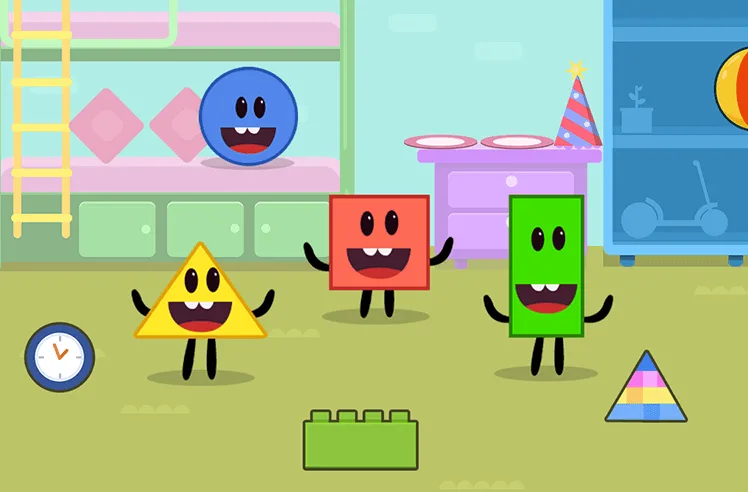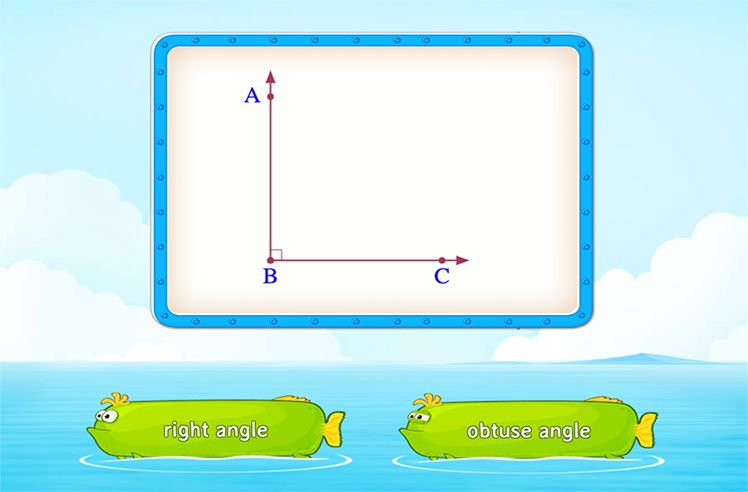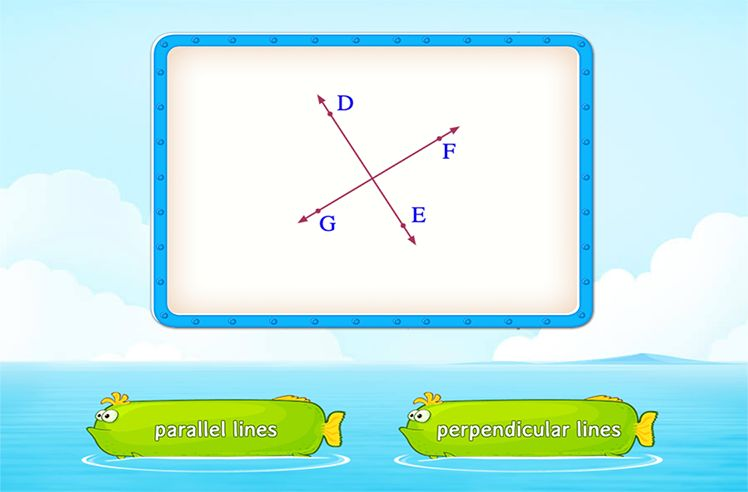# Litres To Milliliters

» Litres To Milliliters

Liters and milliliters are metric units that can measure volume of a liquid. Volume can be measured using milliliters, centiliters, liters, and kiloliters.

We will talk about the connection between liters and milliliters.

## Defining Liter

A liter is often defined as the basic metric unit of measurement of volume. 1 liter = 1 dm3 (cubic decimeter). You can represent the liter by using the letter l or L.

## Defining Milliliter

Milliliter are used to measure a small amount of liquid. Whereas for a larger quantity of liquid, you can use liters. 1 liter = 1000 milliliters or 1 ml = 0.001 l. You can represent milliliter as ml or mL.

## Converting Liters to Milliliters

Now, we know that one liter is equal to 1000 milliliters. So, to convert liters to milliliters or milliliters to liters, we either multiply or divide the number by 1000.

For converting a bigger unit (liter) to a smaller one (milliliter), we multiply the value by 1000. For example: if you want to convert 7 liters to milliliters. The answer will be 7 x 1000 = 7000 ml. In simple words, you can say that seven liters is same as 7000 milliliters.

But, if the case is reversed, i.e., we have to convert from smaller units (milliliter) to bigger units (liter), we divide. We should remember that we should divide the amount by 1000 to convert milliliters to liters.

Consider an example: Convert 5000 milliliters to liters. The answer, in this case, would be 5000 ÷ 1000 = 5 liters. Therefore, 5000 milliliters is same as 5 liters.

## Solved Examples

Question 1: Compare using <, > or =.

1. 22 l  ___  220000 ml
2. 43000 ml  ___  43 l
3. 13 l  ___  3430 ml
4. 8 l  ___  800 ml

1. <

22 l = (22 x 1000) ml = 22000 ml

22000 ml < 220000 ml

1. =

43000 ml = (43000 ÷ 1000) l = 43 l

43 l = 43 l

1. <

13 l = (13 x 1000) ml = 13000 ml

13000 ml < 13430 ml

1. >

8 l = (8 x 1000) ml = 8000 ml

8000 ml > 800 ml

Question 2: Sania uses 3000 ml of detergent in a month. How many liters of detergent is used?

Here, you have to convert smaller unit to larger unit. So, when converting milliliters into liters we need to divide the value by 1000.

3000 ÷ 1000 = 3

Therefore Sania uses 3 l of detergent in a month.

Question 3: A motorcycle has a small petrol tank and can only hold 10 liters of petrol. What is the amount in milliliters?

Answer: Here, you can see that you have to convert the larger unit into the smaller one. So, when converting liters into milliliters, simply multiply the value by 1000.

10 x 1000 = 10000

Therefore, the tank will hold 10000 ml of petrol.

Question 4: A shop sells a water with 25000 ml capacity. What would be the capacity if expressed in liters?

Answer: Here, if you see, we have to convert a smaller unit into a bigger one that is liters. In this question, you would divide the value by 1000. So, the answer would be 25000 ÷ 1000, or 25 liters.

## Practice Problems

Conclusion

The volume of an object can be measured using liters and milliliters. Learning about this conversion is an essential part of measurement. If you want to learn more similar topics, visit SplashLearn.

1000 milliliters makes one liter.

No, 1 l = 1000 ml and 500 ml = 0.5 l.

No, you cannot use a liter as the unit of weight as it is used for measuring volume. Liters measure the space any liquid occupies, not the weight.

You cannot convert from kilogram to liter because kg is used for weight, and liter is used for volume.Shapes

Play NowAngles

Play NowParallel and Perpendicular Lines

Play Now